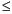Functions and CALL Routines

# GAMINV Function

Returns a quantile from the gamma distribution.
 Category: Quantile

## Syntax

 GAMINV(p,a)

### Arguments

p

is a numeric probability.

 Range: 0p < 1
a

is a numeric shape parameter.

 Range: a > 0

The GAMINV function returns the pth quantile from the gamma distribution, with shape parameter a. The probability that an observation from a gamma distribution is less than or equal to the returned quantile is p.

Note:   GAMINV is the inverse of the PROBGAM function.SAS Statements Results
`q1=gaminv(0.5,9);`
`8.6689511844`
`q2=gaminv(0.1,2.1);`
`0.5841932369`

 Functions:Previous Page | Next Page | Top of Page